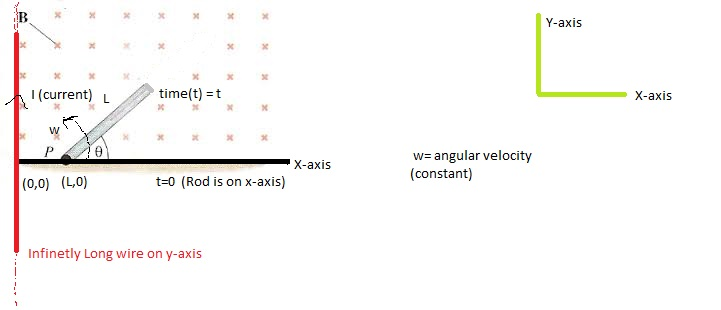# Rotation + Magnetism = RotatismConsider an Thin Conducting Rod of Length L which start's Rotating about an fixed Point P(L,0) in X-Y plane. Initially Rod is on X-axis at an distance of L from origin.(As shown)

There is an infinitely long wire on entire Y-axis. And current is flowing Through this wire is ${ I }_{ 0 }$

This Rod is rotated By an external agent with Constant angular velocity $\omega$. Then Find the Magnitude of Motional EMF develop between the Point 'P' and centre of the rod at the instant when rod is rotated by an angle $\theta ={ 60 }^{ 0 }$

Details and Assumptions

$\bullet$ Long wire and Conducting Rod are Placed in X-Y plane.

$\bullet$ Rod is Rotated By an external agent so that it can definitely move with constant angular velocity.

$\bullet$ EMF means electro motive Force.

Use following data

$\bullet { I }_{ 0 }\quad =4{ \times 10 }^{ 7 }\quad Amp.\\ \bullet \omega =4\quad rad/s\\ \bullet L=4\quad m$.

×